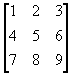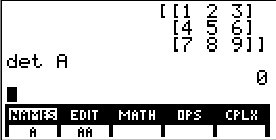# Knowledge Base

## Solution 10350: Finding the Determinant and Inverse of a Matrix on the TI-85 or TI-86.

### How do I find the determinant and inverse of a matrix on the TI-85 or TI-86?

The example below will demonstrate how to find the determinant of a matrix on the TI-85 or TI-86.

Example: Using the data represented here in matrix A.

A =Solution:

To enter the matrix,
• Press [2nd] [MATRX] [F2] to enter the matrix editor
• Input [A] press [ENTER]
• Input the dimensions of the matrix (3 [ENTER] 3 [ENTER])
• Input the values for the elements of the matrix pressing [ENTER] after each entry